# Logic gate

"Discrete logic" redirects here. For discrete circuitry, see Discrete circuit.

In electronics, a logic gate is an idealized or physical device implementing a Boolean function; that is, it performs a logical operation on one or more logical inputs, and produces a single logical output. Depending on the context, the term may refer to an ideal logic gate, one that has for instance zero rise time and unlimited fan-out, or it may refer to a non-ideal physical device (see Ideal and real op-amps for comparison).

Logic gates are primarily implemented using diodes or transistors acting as electronic switches, but can also be constructed using vacuum tubes, electromagnetic relays (relay logic), fluidic logic, pneumatic logic, optics, molecules, or even mechanical elements. With amplification, logic gates can be cascaded in the same way that Boolean functions can be composed, allowing the construction of a physical model of all of Boolean logic, and therefore, all of the algorithms and mathematics that can be described with Boolean logic.

Logic circuits include such devices as multiplexers, registers, arithmetic logic units (ALUs), and computer memory, all the way up through complete microprocessors, which may contain more than 100 million gates. In modern practice, most gates are made from field-effect transistors (FETs), particularly MOSFETs (metal–oxide–semiconductor field-effect transistors).

Compound logic gates AND-OR-Invert (AOI) and OR-AND-Invert (OAI) are often employed in circuit design because their construction using MOSFETs is simpler and more efficient than the sum of the individual gates.

In reversible logic, Toffoli gates are used.

## Electronic gates

Main article: Logic family

To build a functionally complete logic system, relays, valves (vacuum tubes), or transistors can be used. The simplest family of logic gates using bipolar transistors is called resistor-transistor logic (RTL). Unlike simple diode logic gates (which do not have a gain element), RTL gates can be cascaded indefinitely to produce more complex logic functions. RTL gates were used in early integrated circuits. For higher speed and better density, the resistors used in RTL were replaced by diodes resulting in diode-transistor logic (DTL). Transistor-transistor logic (TTL) then supplanted DTL. As integrated circuits became more complex, bipolar transistors were replaced with smaller field-effect transistors (MOSFETs); see PMOS and NMOS. To reduce power consumption still further, most contemporary chip implementations of digital systems now use CMOS logic. CMOS uses complementary (both n-channel and p-channel) MOSFET devices to achieve a high speed with low power dissipation.

For small-scale logic, designers now use prefabricated logic gates from families of devices such as the TTL 7400 series by Texas Instruments, the CMOS 4000 series by RCA, and their more recent descendants. Increasingly, these fixed-function logic gates are being replaced by programmable logic devices, which allow designers to pack a large number of mixed logic gates into a single integrated circuit. The field-programmable nature of programmable logic devices such as FPGAs has reduced the 'hard' property of hardware; it is now possible to change the logic design of a hardware system by reprogramming some of its components, thus allowing the features or function of a hardware implementation of a logic system to be changed.

Other types of logic gates include, but are not limited to 

 Tunnel diode logic TDL Exactly the same as Diode Logic but can perform at a faster speed. Diode logic Neon Logic NL Uses neon bulbs or 3 element neon trigger tubes to perform logic. Core Diode Logic CDL Performed by semiconductor diodes and small ferrite toroidal cores for moderate speed and moderate power level. 4Layer Device Logic 4LDL Uses thyristors and SCR’s to perform logic operations where high current and or high voltages are required. Direct Coupled Transistor Logic DCTL Uses transistors switching between bottom saturated and tunnel states to perform logic, depends upon use of transistors with carefully controlled parameters. Economical but tends to be susceptible to noise because of the lower voltage levels employed. Often considered to be the father to modern TTL logic. Direct-coupled transistor logic Current Mode Logic CML Uses transistors to perform logic but biasing is from constant current sources to prevent saturation and allow extremely fast switching. Has high noise immunity despite fairly low logic levels. Current-mode logic Diode logic DL Diode logic

Electronic logic gates differ significantly from their relay-and-switch equivalents. They are much faster, consume much less power, and are much smaller (all by a factor of a million or more in most cases). Also, there is a fundamental structural difference. The switch circuit creates a continuous metallic path for current to flow (in either direction) between its input and its output. The semiconductor logic gate, on the other hand, acts as a high-gain voltage amplifier, which sinks a tiny current at its input and produces a low-impedance voltage at its output. It is not possible for current to flow between the output and the input of a semiconductor logic gate.

Another important advantage of standardized integrated circuit logic families, such as the 7400 and 4000 families, is that they can be cascaded. This means that the output of one gate can be wired to the inputs of one or several other gates, and so on. Systems with varying degrees of complexity can be built without great concern of the designer for the internal workings of the gates, provided the limitations of each integrated circuit are considered.

The output of one gate can only drive a finite number of inputs to other gates, a number called the 'fanout limit'. Also, there is always a delay, called the 'propagation delay', from a change in input of a gate to the corresponding change in its output. When gates are cascaded, the total propagation delay is approximately the sum of the individual delays, an effect which can become a problem in high-speed circuits. Additional delay can be caused when a large number of inputs are connected to an output, due to the distributed capacitance of all the inputs and wiring and the finite amount of current that each output can provide.

## History and development

The binary number system was refined by Gottfried Wilhelm Leibniz (published in 1705) and he also established that by using the binary system, the principles of arithmetic and logic could be combined.

In an 1886 letter, Charles Sanders Peirce described how logical operations could be carried out by electrical switching circuits. Eventually, vacuum tubes replaced relays for logic operations. Lee De Forest's modification, in 1907, of the Fleming valve can be used as an AND logic gate. Ludwig Wittgenstein introduced a version of the 16-row truth table as proposition 5.101 of Tractatus Logico-Philosophicus (1921). Walther Bothe, inventor of the coincidence circuit, got part of the 1954 Nobel Prize in physics, for the first modern electronic AND gate in 1924. Konrad Zuse designed and built electromechanical logic gates for his computer Z1 (from 1935–38).

Claude E. Shannon introduced the use of Boolean algebra in the analysis and design of switching circuits in 1937. Using this property of electrical switches to implement logic is the fundamental concept that underlies all electronic digital computers. Shannon's work became the foundation of digital circuit design, as it became widely known in the electrical engineering community during and after World War II. The theoretical rigor of Shannon's work superseded the ad hoc methods that had prevailed previously.

Active research is taking place in molecular logic gates.

## Symbols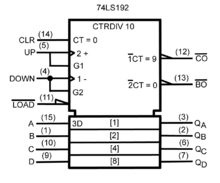A synchronous 4-bit up/down decade counter symbol (74LS192) in accordance with ANSI/IEEE Std. 91-1984 and IEC Publication 60617-12.

There are two sets of symbols for elementary logic gates in common use, both defined in ANSI/IEEE Std 91-1984 and its supplement ANSI/IEEE Std 91a-1991. The "distinctive shape" set, based on traditional schematics, is used for simple drawings, and derives from MIL-STD-806 of the 1950s and 1960s. It is sometimes unofficially described as "military", reflecting its origin. The "rectangular shape" set, based on ANSI Y32.14 and other early industry standards, as later refined by IEEE and IEC, has rectangular outlines for all types of gate and allows representation of a much wider range of devices than is possible with the traditional symbols. The IEC standard, IEC 60617-12, has been adopted by other standards, such as EN 60617-12:1999 in Europe, BS EN 60617-12:1999 in the United Kingdom, and DIN EN 60617-12:1998 in Germany.

The mutual goal of IEEE Std 91-1984 and IEC 60617-12 was to provide a uniform method of describing the complex logic functions of digital circuits with schematic symbols. These functions were more complex than simple AND and OR gates. They could be medium scale circuits such as a 4-bit counter to a large scale circuit such as a microprocessor.

IEC 617-12 and its successor IEC 60617-12 do not explicitly show the "distinctive shape" symbols, but do not prohibit them. These are, however, shown in ANSI/IEEE 91 (and 91a) with this note: "The distinctive-shape symbol is, according to IEC Publication 617, Part 12, not preferred, but is not considered to be in contradiction to that standard." IEC 60617-12 correspondingly contains the note (Section 2.1) "Although non-preferred, the use of other symbols recognized by official national standards, that is distinctive shapes in place of symbols [list of basic gates], shall not be considered to be in contradiction with this standard. Usage of these other symbols in combination to form complex symbols (for example, use as embedded symbols) is discouraged." This compromise was reached between the respective IEEE and IEC working groups to permit the IEEE and IEC standards to be in mutual compliance with one another.

A third style of symbols was in use in Europe and is still widely used in European academia. See the column "DIN 40700" in the table in the German Wikipedia.

In the 1980s, schematics were the predominant method to design both circuit boards and custom ICs known as gate arrays. Today custom ICs and the field-programmable gate array are typically designed with Hardware Description Languages (HDL) such as Verilog or VHDL.

Type Distinctive shape
(IEEE Std 91/91a-1991)
Rectangular shape
(IEEE Std 91/91a-1991
IEC 60617-12 : 1997)
Boolean algebra between A & B Truth table
AND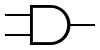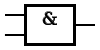INPUT OUTPUT A B A AND B 0 0 0 0 1 0 1 0 0 1 1 1
OR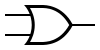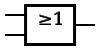INPUT OUTPUT A B A OR B 0 0 0 0 1 1 1 0 1 1 1 1
NOT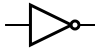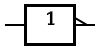INPUT OUTPUT A NOT A 0 1 1 0
In electronics a NOT gate is more commonly called an inverter. The circle on the symbol is called a bubble, and is used in logic diagrams to indicate a logic negation between the external logic state and the internal logic state (1 to 0 or vice versa). On a circuit diagram it must be accompanied by a statement asserting that the positive logic convention or negative logic convention is being used (high voltage level = 1 or high voltage level = 0, respectively). The wedge is used in circuit diagrams to directly indicate an active-low (high voltage level = 0) input or output without requiring a uniform convention throughout the circuit diagram. This is called Direct Polarity Indication. See IEEE Std 91/91A and IEC 60617-12. Both the bubble and the wedge can be used on distinctive-shape and rectangular-shape symbols on circuit diagrams, depending on the logic convention used. On pure logic diagrams, only the bubble is meaningful.
NAND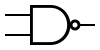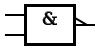INPUT OUTPUT A B A NAND B 0 0 1 0 1 1 1 0 1 1 1 0
NOR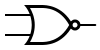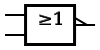INPUT OUTPUT A B A NOR B 0 0 1 0 1 0 1 0 0 1 1 0
XOR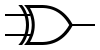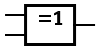INPUT OUTPUT A B A XOR B 0 0 0 0 1 1 1 0 1 1 1 0
The output of a two input exclusive-OR is true only when the two input values are different, and false if they are equal, regardless of the value. If there are more than two inputs, the output of the distinctive-shape symbol is undefined. The output of the rectangular-shaped symbol is true if the number of true inputs is exactly one or exactly the number following the "=" in the qualifying symbol. In practice, these gates are built from combinations of simpler logic gates.
XNOR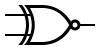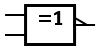INPUT OUTPUT A B A XNOR B 0 0 1 0 1 0 1 0 0 1 1 1

## Universal logic gates

For more details on the theoretical basis, see Functional completeness.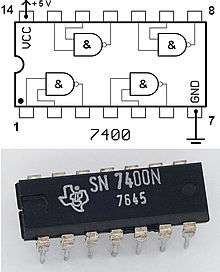The 7400 chip, containing four NANDs. The two additional pins supply power (+5 V) and connect the ground.

Charles Sanders Peirce (during 1880–81) showed that NOR gates alone (or alternatively NAND gates alone) can be used to reproduce the functions of all the other logic gates, but his work on it was unpublished until 1933. The first published proof was by Henry M. Sheffer in 1913, so the NAND logical operation is sometimes called Sheffer stroke; the logical NOR is sometimes called Peirce's arrow. Consequently, these gates are sometimes called universal logic gates.

## De Morgan equivalent symbols

By use of De Morgan's laws, an AND function is identical to an OR function with negated inputs and outputs. Likewise, an OR function is identical to an AND function with negated inputs and outputs. A NAND gate is equivalent to an OR gate with negated inputs, and a NOR gate is equivalent to an AND gate with negated inputs.

This leads to an alternative set of symbols for basic gates that use the opposite core symbol (AND or OR) but with the inputs and outputs negated. Use of these alternative symbols can make logic circuit diagrams much clearer and help to show accidental connection of an active high output to an active low input or vice versa. Any connection that has logic negations at both ends can be replaced by a negationless connection and a suitable change of gate or vice versa. Any connection that has a negation at one end and no negation at the other can be made easier to interpret by instead using the De Morgan equivalent symbol at either of the two ends. When negation or polarity indicators on both ends of a connection match, there is no logic negation in that path (effectively, bubbles "cancel"), making it easier to follow logic states from one symbol to the next. This is commonly seen in real logic diagrams - thus the reader must not get into the habit of associating the shapes exclusively as OR or AND shapes, but also take into account the bubbles at both inputs and outputs in order to determine the "true" logic function indicated.

A De Morgan symbol can show more clearly a gate's primary logical purpose and the polarity of its nodes that are considered in the "signaled" (active, on) state. Consider the simplified case where a two-input NAND gate is used to drive a motor when either of its inputs are brought low by a switch. The "signaled" state (motor on) occurs when either one OR the other switch is on. Unlike a regular NAND symbol, which suggests AND logic, the De Morgan version, a two negative-input OR gate, correctly shows that OR is of interest. The regular NAND symbol has a bubble at the output and none at the inputs (the opposite of the states that will turn the motor on), but the De Morgan symbol shows both inputs and output in the polarity that will drive the motor.

De Morgan's theorem is most commonly used to implement logic gates as combinations of only NAND gates, or as combinations of only NOR gates, for economic reasons.

## Data storage

Main article: Sequential logic

Logic gates can also be used to store data. A storage element can be constructed by connecting several gates in a "latch" circuit. More complicated designs that use clock signals and that change only on a rising or falling edge of the clock are called edge-triggered "flip-flops". Formally, a flip-flop is called a bistable circuit, because it has two stable states which it can maintain indefinitely. The combination of multiple flip-flops in parallel, to store a multiple-bit value, is known as a register. When using any of these gate setups the overall system has memory; it is then called a sequential logic system since its output can be influenced by its previous state(s), i.e. by the sequence of input states. In contrast, the output from combinatorial logic is purely a combination of its present inputs, unaffected by the previous input and output states.

These logic circuits are known as computer memory. They vary in performance, based on factors of speed, complexity, and reliability of storage, and many different types of designs are used based on the application.

## Three-state logic gatesA tristate buffer can be thought of as a switch. If B is on, the switch is closed. If B is off, the switch is open.
Main article: Tri-state buffer

A three-state logic gate is a type of logic gate that can have three different outputs: high (H), low (L) and high-impedance (Z). The high-impedance state plays no role in the logic, which is strictly binary. These devices are used on buses of the CPU to allow multiple chips to send data. A group of three-states driving a line with a suitable control circuit is basically equivalent to a multiplexer, which may be physically distributed over separate devices or plug-in cards.

In electronics, a high output would mean the output is sourcing current from the positive power terminal (positive voltage). A low output would mean the output is sinking current to the negative power terminal (zero voltage). High impedance would mean that the output is effectively disconnected from the circuit.

## Implementations

Since the 1990s, most logic gates are made in CMOS technology (i.e. NMOS and PMOS transistors are used). Often millions of logic gates are packaged in a single integrated circuit.

There are several logic families with different characteristics (power consumption, speed, cost, size) such as: RDL (resistor-diode logic), RTL (resistor-transistor logic), DTL (diode-transistor logic), TTL (transistor-transistor logic) and CMOS (complementary metal oxide semiconductor). There are also sub-variants, e.g. standard CMOS logic vs. advanced types using still CMOS technology, but with some optimizations for avoiding loss of speed due to slower PMOS transistors.

Non-electronic implementations are varied, though few of them are used in practical applications. Many early electromechanical digital computers, such as the Harvard Mark I, were built from relay logic gates, using electro-mechanical relays. Logic gates can be made using pneumatic devices, such as the Sorteberg relay or mechanical logic gates, including on a molecular scale. Logic gates have been made out of DNA (see DNA nanotechnology) and used to create a computer called MAYA (see MAYA II). Logic gates can be made from quantum mechanical effects (though quantum computing usually diverges from boolean design). Photonic logic gates use non-linear optical effects.

In principle any method that leads to a gate that is functionally complete (for example, either a NOR or a NAND gate) can be used to make any kind of digital logic circuit. Note that the use of 3-state logic for bus systems is not needed, and can be replaced by digital multiplexers, which can be built using only simple logic gates (such as NAND gates, NOR gates, or AND and OR gates).

## References

1. Jaeger, Microelectronic Circuit Design, McGraw-Hill 1997, ISBN 0-07-032482-4, pp. 226-233
2. Tinder, Richard F. (2000). Engineering digital design: Revised Second Edition. pp. 317–319. ISBN 0-12-691295-5. Retrieved 2008-07-04.
3. Rowe, Jim. "Circuit Logic - Why and How" (December 1966). Electronics Australia.
4. Peirce, C. S., "Letter, Peirce to A. Marquand", dated 1886, Writings of Charles S. Peirce, v. 5, 1993, pp. 541–3. Google Preview. See Burks, Arthur W., "Review: Charles S. Peirce, The new elements of mathematics", Bulletin of the American Mathematical Society v. 84, n. 5 (1978), pp. 913–18, see 917. PDF Eprint.
5. Overview of IEEE Standard 91-1984 Explanation of Logic Symbols, Doc. No. SDYZ001A, Texas Instruments Semiconductor Group, 1996
6. Peirce, C. S. (manuscript winter of 1880–81), "A Boolean Algebra with One Constant", published 1933 in Collected Papers v. 4, paragraphs 12–20. Reprinted 1989 in Writings of Charles S. Peirce v. 4, pp. 218-21, Google Preview. See Roberts, Don D. (2009), The Existential Graphs of Charles S. Peirce, p. 131.
7. Hans Kleine Büning; Theodor Lettmann (1999). Propositional logic: deduction and algorithms. Cambridge University Press. p. 2. ISBN 978-0-521-63017-7.
8. John Bird (2007). Engineering mathematics. Newnes. p. 532. ISBN 978-0-7506-8555-9.
9. Mechanical Logic gates (focused on molecular scale)
10. DNA Logic gates